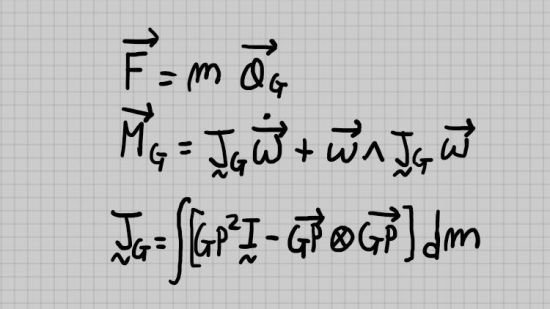﻿ Advanced Rigid Body Mechanics in Three Dimensions » GFXhome

» » Advanced Rigid Body Mechanics in Three Dimensions
Information of news
20-06-2021, 09:56

### Advanced Rigid Body Mechanics in Three Dimensions

Category: TutorialsMP4 | Video: h264, 1280x720 | Audio: AAC, 44.1 KHz, 2 Ch
Genre: eLearning | Language: English + srt | Duration: 22 lectures (4h 5m) | Size: 1.81 GB

Mathematical intuition behind the fundamental equations in rigid body mechanics
What you'll learn:
How to derive the fundamental equations on rigid body kinematics and dynamics
How to derive energy conservation from Newton's laws (Virtual Work Theorem)
Fundamental properties of the angular velocity of a rigid body

Requirements
Newton laws of motion
Calculus, Multivariable Calculus (especially: derivatives, multiple integrals)
Vectors, dot products, cross products

Description
This is a course on the fundamental equations and concepts which revolve around rigid bodies. All the equations are derived with detailed explanations, but the following mathematical prerequisites are needed: vectors, dot and cross products, some linear algebra (matrices, determinants, eingenvectors, eigenvalues), some calculus (especially: derivatives, volume integrals). As regards the physics of the course, the only prerequisite is the knowledge of Newton's equations. In fact, these equations constitute the physical foundation of the course, since the rigid body mechanics are constructed from point-particle dynamics (i.e. the law: F=ma, where F is the total force acting on a point-particle, a is the acceleration, m is the mass, is postulated to be true for point-particles).

In the course, the inertia matrix is derived, which will appear in the equation of moments, as well as in the expression of the kinetic energy of a rigid body. The concept of angular velocity is also derived, and it will be shown that it is unique. Other important formulae regarding kinematics are derived, which will relate velocities and accelerations of generic points of a rigid body.

In kinematics, we will derive Chasles' theorem, or Mozzi-Chasles' theorem, which says that the most general rigid body displacement can be produced by a translation along a line (called Mozzi axis), in conjunction with a rotation about the same line.

Who this course is for
Students who would like to develop mathematical intution to tackle problems about rigid body systems.Site BBcode/HTML Code:
Dear visitor, you went to the site as unregistered user.